Tip: Other languages are Google-Translated. You can visit the English version of this link.orRegisteror

## How to calculate interest payments per period or total with Excel formulas?

#### Calculate monthly interest payments on a credit card in Excel

For example, you sign a credit card installment agreement, and you will pay your bill of \$2,000 in 12 months with annual interest rate of 9.6%. In this example, you can apply the IPMT function to calculate the interest payment per month easily.

1. According to the information of your credit card bill, you can list the data in Excel as below screenshot: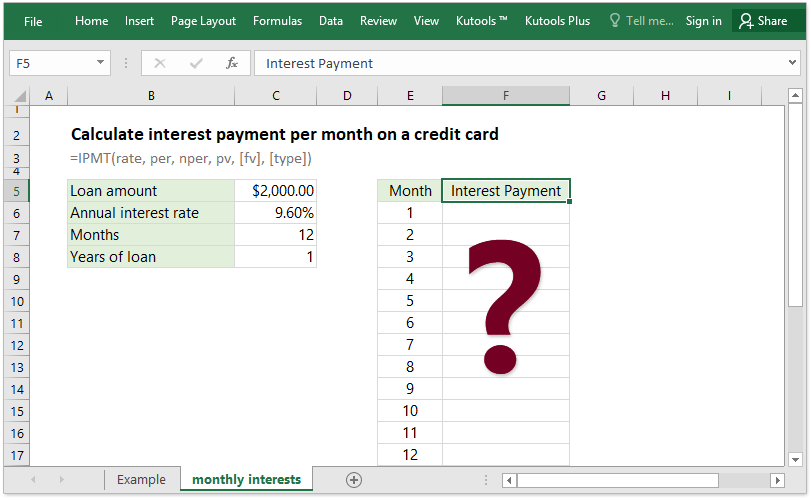2. In the Cell F6, please type below formula, and press the Enter key. See screenshot:

=IPMT(\$C\$6/\$C\$7,E6,\$C\$7*\$C\$8, \$C\$5)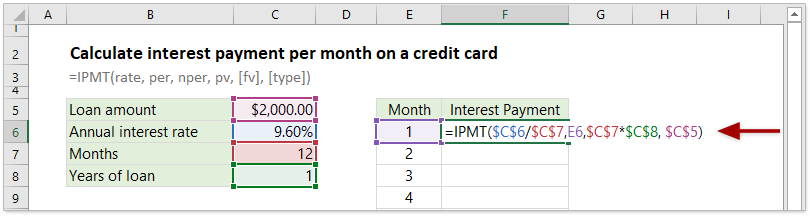3. Keep the formula cell F6 selected, and drag its AutoFill handle down to the cells you will apply this formula.

Now the interest payments per month are figured out at once. See screenshot: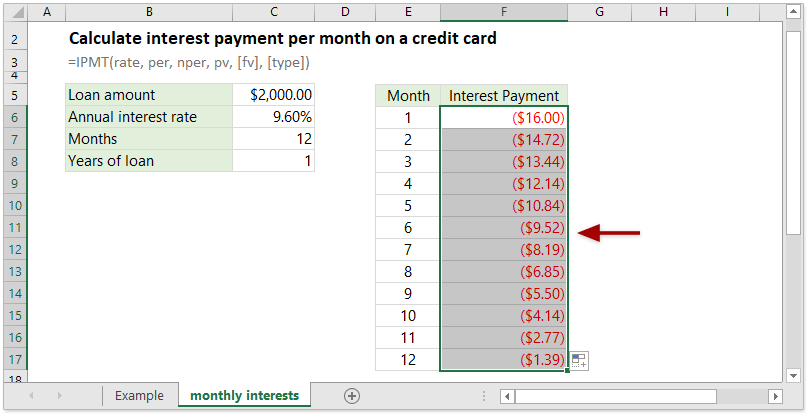#### Calculate quarterly interest payments for a loan in Excel

Supposing you have a loan of \$10,000 from your bank, and the loan rate is 8.5%. From now on you need to pay back the loan in quarterly installment in 2 years. Now you can also apply the IPMT function to calculate the interest payment per quarter easily in Excel.

1. According to the information of your loan, you can list the data in Excel as below screenshot: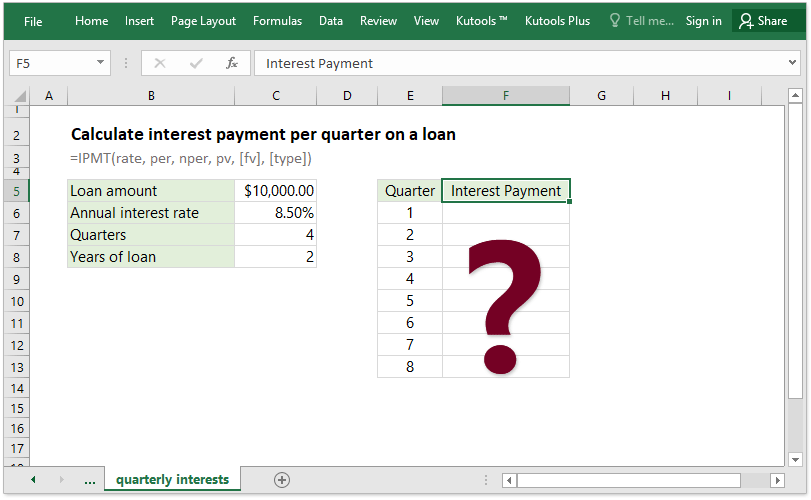2. In the Cell F6, please type below formula, and press the Enter key.

=IPMT(\$C\$6/\$C\$7,E6,\$C\$7*\$C\$8, \$C\$5)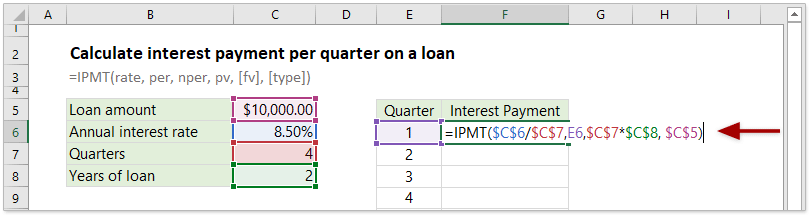3. Keep the formula cell F6 selected, and drag its AutoFill handle down to the range as you need.

Now the interest payment of each quarter in the whole life of loan is figured out at once. See screenshot: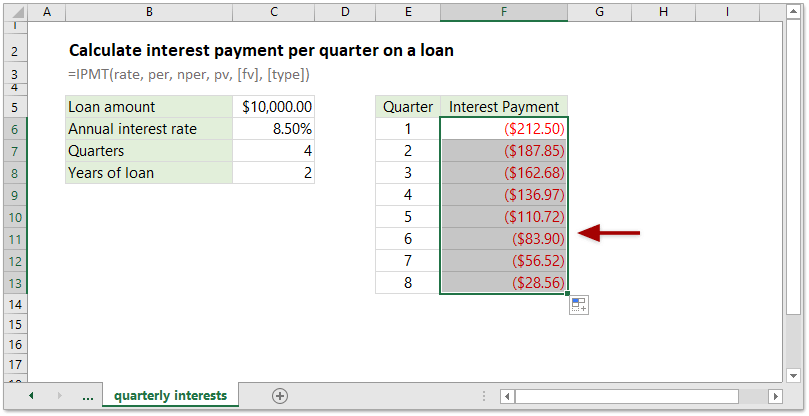#### Calculate semi-annual interest payments on a car loan in Excel

Assume you are going to buy a car with a bank loan. The amount of this bank loan is \$50,000, its interest rate is 6.4%, you will repay the loan twice at the end of every half year, and the life of this bank loan is 5 years. In this case, you can easily figure out how much interest you will pay semi-annually easily with the IPMT function in Excel.

1. According to the information of your car loan, you can list the data in Excel as below screenshot: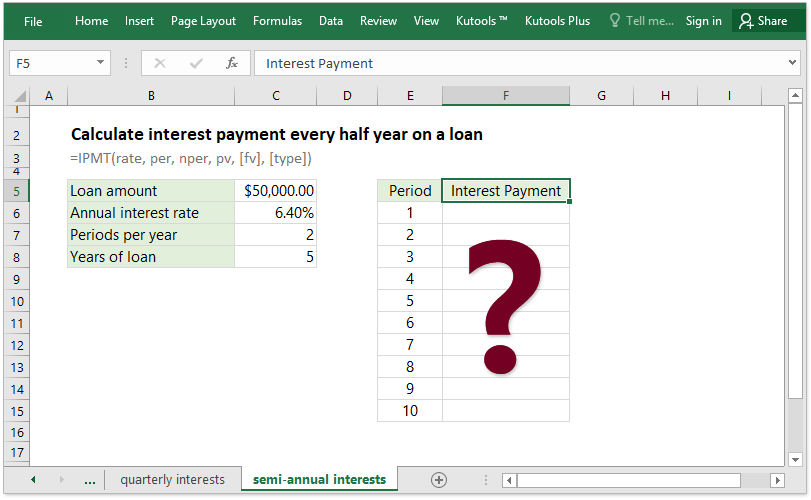2. In the Cell F6, enter below formula, and press the Enter key.

=IPMT(\$C\$6/\$C\$7,E6,\$C\$7*\$C\$8, -\$C\$5)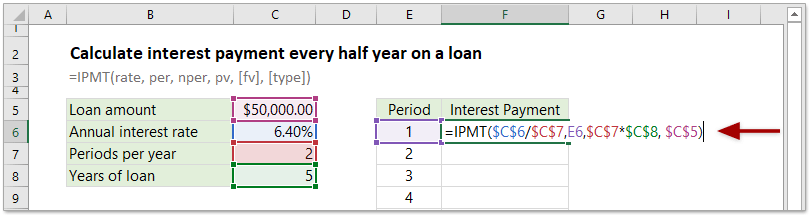3. Drag the AutoFill handle of this formula cell down to the range as you need.

Now the interest payment of every half year is calculated immediately. See screenshot: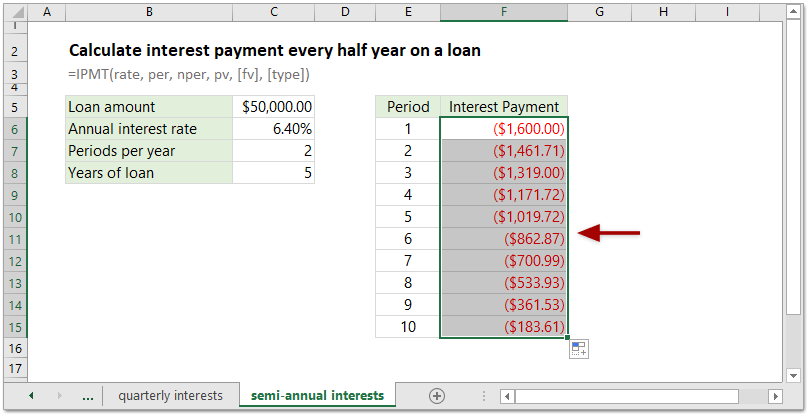#### Calculate total interest paid on a loan in Excel

Sometimes, you may want to calculate the total interest paid on a loan. For periodic, constant payments and constant interest rate, you can apply the IPMT function to figure out the interest payment for every period, and then apply the Sum function to sum up these interest payments, or apply the CUMIPMT function to get the total interest paid on a loan directly in Excel.

For example, you have a loan of \$5,000 with annual interest rate of 8.00%. Now you need to repay it monthly in half year. You can figure out the total interest paid as follows:

1. List your loan data in Excel as below screenshot shown: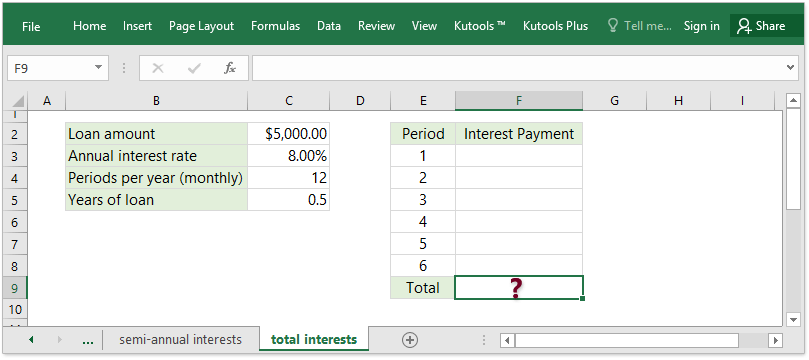2. In Cell F3, type in the formula, and drag the formula cell’s AutoFill handle down the range as you need.

=IPMT(\$C\$3/\$C\$4,E3,\$C\$4*\$C\$5, \$C\$2)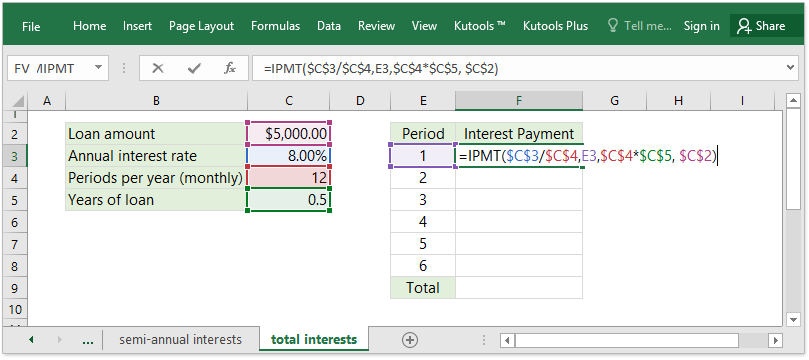3. In the Cell F9, type in the formula =SUM(F3:F8), and press the Enter key.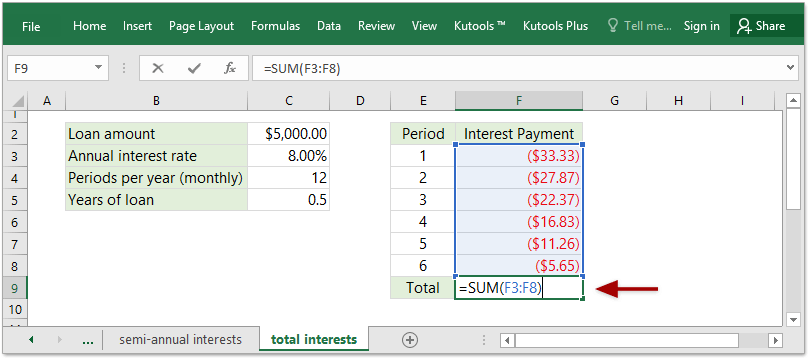Now you will get the total amount of interest payments for the loan.

Note: You can also apply the CUMIPMT function to calculate the total interest payments.

=CUMIPMT(C3/C4,C4*C5,C2,1,6,0)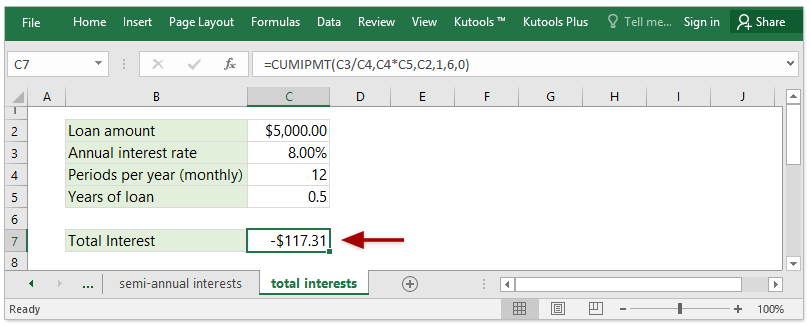In above formula, C3/C4 will calculate the monthly interest rate, C4*C5 will get the total number of periods, C2 is the loan amount you received, 1 means the first period you will pay back the loan, 6 indicates the last period (there are 6 periods in total), and 0 indicates you repay at the end of every period.

### Excel Productivity Tools

Would you like to complete your daily work quickly and perfectly? Kutools for Excel brings 300 powerful advanced features (Combine workbooks, sum by color, split cell contents, convert date, and so on...) and save 70% time.

• Designed for 1500 work scenarios, helps you solve 80% Excel problems.
• Reduce thousands of keyboard and mouse clicks every day, relieve your tired eyes and hands.
• Become an Excel expert in 3 minutes. No longer need to remember any painful formulas and VBA codes.
• 60-day unlimited free trial. 60-day money back guarantee. Free upgrade and support for 2 years.

#### Brings Efficient Tabs to Office (include Excel), Just Like Chrome, Firefox, And New IE

• Office Tab Increases your productivity by 50% when viewing and editing multiple documents.
• Reduce hundreds of mouse clicks for you every day, say goodbye to mouse hand.
• One second to switch between dozens of open documents!Say something here...
symbols left.